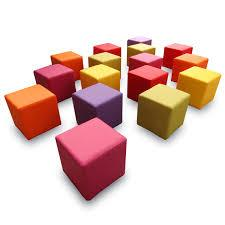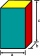# Cubes into cuboid

How many 12 centimeter cubes fit into the block (cuboid) with 6dm, 8.4dm, and 4.8?

n =  140

### Step-by-step explanation:Did you find an error or inaccuracy? Feel free to write us. Thank you!Tips to related online calculators
Do you know the volume and unit volume, and want to convert volume units?

#### You need to know the following knowledge to solve this word math problem:

We encourage you to watch this tutorial video on this math problem:

## Related math problems and questions:

• AquariumCan 30 liters of water fit in a cuboid aquarium with dimensions a = 3dm b = 6dm c = 5dm?
• Water blockA block with a 50 cm2 base is filled with water 5 cm under the edge. How many can sugar cubes with 2 cm edge be thrown into a container that overflow water?
• Identical cubesFrom the smallest number of identical cubes whose edge length is expressed by a natural number, can we build a block with dimensions 12dm x 16dm x 20dm?
• Into boxHow many cubes with an edge of 2.5 cm fit into a box measuring 11.6 cm; 8.9 cm and 13.75 cm?
• Swimming poolThe swimming pool has the shape of a block with dimensions of 70dm, 25m, 200cm. How many hl of water can fit into the pool?
• The woodenThe wooden block measures 12 cm, 24 cm, and 30 cm. Peter wants to cut it into several identical cubes. At least how many cubes can he get?
• CubesHow many cubes with an edge length of 5 cm may fit in the cube with an inner edge of 0.4 m maximally?
• Prism 4 sidesFind the surface area and volume four-sided prism high 10cm if its base is a rectangle measuring 8 cm and 1.2dm
• Cuboids in cubeHow many cuboids with dimensions of 6 cm, 8 cm and 12 cm can fit into a cube with side 96 centimeters?
• Edges of the cuboidFind the length of the edges of the cuboid, which has the following dimensions: width is 0.4 m; the height is 5.8 dm and the block can hold 81.2 liters of fluid.
• Wooden blockA cuboid-shaped wooden block has 6 cm length, 4 cm breadth, and 1 cm height. Two faces measuring 4 cm x 1 cm are colored in black. Two faces measuring 6 cm x 1 cm are colored in red. Two faces measuring 6 cm x 4 cm are colored in green. The block is divid
• TetrapackHow high should be the milk box in the shape of a prism with base dimensions 8 cm and 8.8 cm if its volume is 1 liter?
• The numberThe number of 1 cm cubes required to make 4 cm cube is?
• Hectoliter into cubeCan 1hl of water fit into a half-meter cube?
• Milk packageMilk is sold in a box with dimensions of 9.5 cm; 16.5 cm and 6.5 cm. Determine the maximum amount of milk that can fit into a box. Coating thickness is negligible.
• Surface areaCalculate the surface area of a four-sides 2-m high prism which base is a rectangle with sides 17 cm and 1.3 dm
• Two cuboidsFind the volume of the cuboidal box whose one edge is: a) 1.4m and b) 2.1 dm.